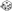General Use Thread - Page 58 - Myth-Weavers

Olbryn casts CLW (prepared) on Nikhil's horse:
Dice Roll: 1d8+5
d8 Results: 8 (Total = 13)

Young Dragon

W4 Will save against Color Spray

Save DC=15
Will Save
Dice Roll: 1D20+6z
d20 Results: 14 (Total = 20)

Gan AoO's with Guisarme

Hit:
Dice Roll: 1d20+8
d20 Results: 13 (Total = 21)

Damage:
Dice Roll: 2d4+17
d4 Results: 4, 2 (Total = 23)

Hit:
Dice Roll: 1d20+8
d20 Results: 4 (Total = 12)

Damage:
Dice Roll: 2d4+17
d4 Results: 3, 4 (Total = 24)

Hit:
Dice Roll: 1d20+8
d20 Results: 20 (Total = 28)

Damage:
Dice Roll: 2d4+17
d4 Results: 3, 2 (Total = 22)

Garçon attacks O2 with bite

Attack
Dice Roll: 1d20+3
d20 Results: 2 (Total = 5)

Damage
Dice Roll: 1d6+3
d6 Results: 2 (Total = 5)

Tibbideau cast magic missile as wizard at O2

Concentration (DC 21)
Dice Roll: 1d20+11
d20 Results: 2 (Total = 13)

Damage
Dice Roll: 3d4+11
d4 Results: 1, 4, 2 (Total = 18)

Gan attack W5:
Hit:
Dice Roll: 1d20+8
d20 Results: 12 (Total = 20)

Damage:
Dice Roll: 2d4+17
d4 Results: 4, 3 (Total = 24)

Gan AoO's with Guisarme:
Hit:
Dice Roll: 1d20+8
d20 Results: 13 (Total = 21)

Damage:
Dice Roll: 2d4+17
d4 Results: 1, 2 (Total = 20)

Hit:
Dice Roll: 1d20+8
d20 Results: 1 (Total = 9)

Damage:
Dice Roll: 2d4+17
d4 Results: 3, 4 (Total = 24)

Hit:
Dice Roll: 1d20+8
d20 Results: 4 (Total = 12)

Damage:
Dice Roll: 2d4+17
d4 Results: 3, 1 (Total = 21)

Dice Roll: 1d20+7+2z
d20 Results: 18 (Total = 27)AoO on W6 (27)
Dice Roll: 2d4+7+2z
d4 Results: 2, 1 (Total = 12)
These dice were omitted, altered, or moved: 2d4+7+2
Original Dice: 2d4+7+2dmg (12)
Dice Roll: 5d6z
d6 Results: 3, 2, 4, 6, 1 (Total = 16)Shocking grasp dmg (16)

Dice Roll: 1d20+7+2-2z
d20 Results: 7 (Total = 14)attack on W6 with PA (14)
Dice Roll: 2d4+7+2+4z
d4 Results: 2, 2 (Total = 15)dmg (15)

Tibbideau escape artist check (immediate action)

Dice Roll: 1d20+11
d20 Results: 9 (Total = 20)